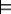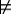### 12.3.2 Interpreting Variables

When a variable appears in a clause, the clause is true in an interpretation only if the clause is true for all possible values of that variable. The variable is said to be universally quantified within the scope of the clause. If a variable X appears in a clause C, then claiming that C is true in an interpretation means that C is true no matter which individual from the domain is denoted by X.

To formally define semantics of variables, a variable assignment, ρ, is a function from the set of variables into the domain D. Thus, a variable assignment assigns an element of the domain to each variable. Given φ and a variable assignment ρ, each term denotes an individual in the domain. If the term is a constant, the individual denoted is given by φ. If the term is a variable, the individual denoted is given by ρ. Given an interpretation and a variable assignment, each atom is either true or false, using the same definition as earlier. Similarly, given an interpretation and a variable assignment, each clause is either true or false.

A clause is true in an interpretation if it is true for all variable assignments. This is called a universal quantification. The variables are said to be universally quantified in the scope of the clause. Thus, a clause is false in an interpretation means there is a variable assignment under which the clause is false. The scope of the variable is the whole clause, which means that the same variable assignment is used for all instances of a variable in a clause.

Example 12.6: The clause
part_of(X,Y) ←in(X,Y).

is false in the interpretation of Example 12.4, because under the variable assignment with X denoting Kim and Y denoting Room 123, the clause's body is true and the clause's head is false.

The clause

in(X,Y) ←part_of(Z,Y) ∧in(X,Z).

is true, because in all variable assignments where the body is true, the head is also true.

Logical consequence is defined as in Section 5.1: ground body g is a logical consequence of KB, written KBg, if g is true in every model of KB.

Example 12.7: Suppose the knowledge base KB is
in(kim,r123).
part_of(r123,cs_building).
in(X,Y) ←
part_of(Z,Y) ∧
in(X,Z).

The interpretation defined in Example 12.4 is a model of KB, because each clause is true in that interpretation.

KBin(kim,r123), because this is stated explicitly in the knowledge base. If every clause of KB is true in an interpretation, then in(kim,r123) must be true in that interpretation.

KBin(kim,r023). The interpretation defined in Example 12.4 is a model of KB, in which in(kim,r023) is false.

KBpart_of(r023,cs_building). Although part_of(r023,cs_building) is true in the interpretation of Example 12.4, there is another model of KB in which part_of(r023,cs_building) is false. In particular, the interpretation which is like the interpretation of Example 12.4, but where
π(part_of)(⟨φ(r023),φ(cs_building)⟩)=false,
is a model of KB in which part_of(r023,cs_building) is false.

KBin(kim,cs_building). If the clauses in KB are true in interpretation I, it must be the case that in(kim,cs_building) is true in I, otherwise there is an instance of the third clause of KB that is false in I - a contradiction to I being a model of KB.

Notice how the semantics treats variables appearing in a clause's body but not in its head (see Example 12.8).

Example 12.8: In Example 12.7, the variable Y in the clause defining in is universally quantified at the level of the clause; thus, the clause is true for all variable assignments. Consider particular values c1 for X and c2 for Y. The clause
in(c1,c2) ←
part_of(Z,c2) ∧
in(c1,Z).

is true for all variable assignments to Z. If there exists a variable assignment c3 for Z such that part_of(Z,c2) ∧in(c1,Z) is true in an interpretation, then in(c1,c2) must be true in that interpretation. Therefore, you can read the last clause of Example 12.7 as "for all X and for all Y, in(X,Y) is true if there exists a Z such that part_of(Z,Y) ∧in(X,Z) is true."

When we want to make the quantification explicit, we write ∀X  p(X), which reads, "for all X, p(X)," to mean p(X) is true for every variable assignment for X. We write exist X  p(X) and read "there exists X such that p(X)" to mean p(X) is true for some variable assignment for X. X is said to be an existentially quantified variable.

The rule P(X) ←Q(X,Y) means

∀X ∀Y (P(X) ←Q(X,Y)),

which is equivalent to

∀X (P(X) ←exist Y Q(X,Y)).

Thus, free variables that only appear in the body are existentially quantified in the scope of the body.

It may seem as though there is something peculiar about talking about the clause being true for cases where it does not make sense.

Example 12.9: Consider the clause
in(cs422,love) ←
part_of(cs422,sky) ∧
in(sky,love).

where cs422 denotes a course, love denotes an abstract concept, and sky denotes the sky. Here, the clause is vacuously true in the intended interpretation according to the truth table for , because the clause's right-hand side is false in the intended interpretation.

As long as whenever the head is non-sensical, the body is also, the rule can never be used to prove anything non-sensical. When checking for the truth of a clause, you must only be concerned with those cases in which the clause's body is true. Using the convention that a clause is true whenever the body is false, even if it does not make sense, makes the semantics simpler and does not cause any problems.